# Calculating Financial Ratios Calculating Financial Ratios Lesson Goals

• Slides: 15Calculating Financial Ratios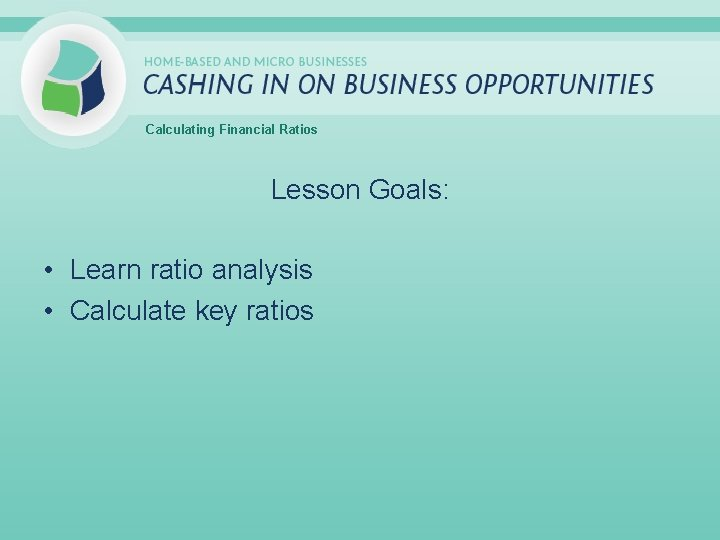Calculating Financial Ratios Lesson Goals: • Learn ratio analysis • Calculate key ratios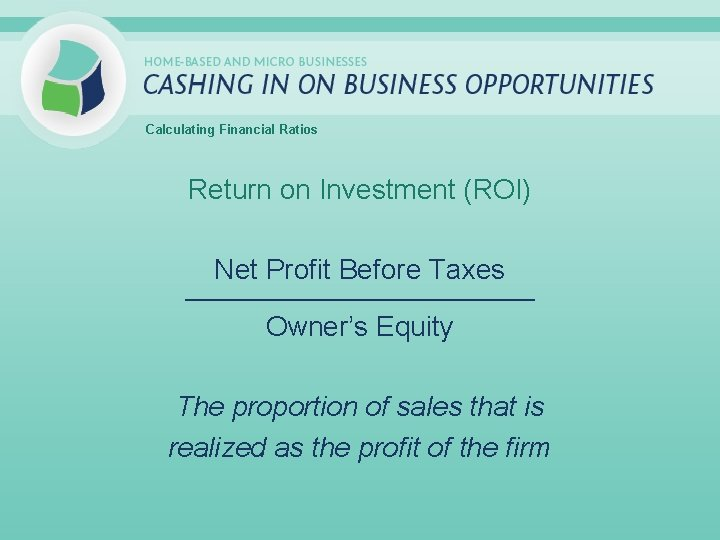Calculating Financial Ratios Return on Investment (ROI) Net Profit Before Taxes _____________________________ Owner’s Equity The proportion of sales that is realized as the profit of the firm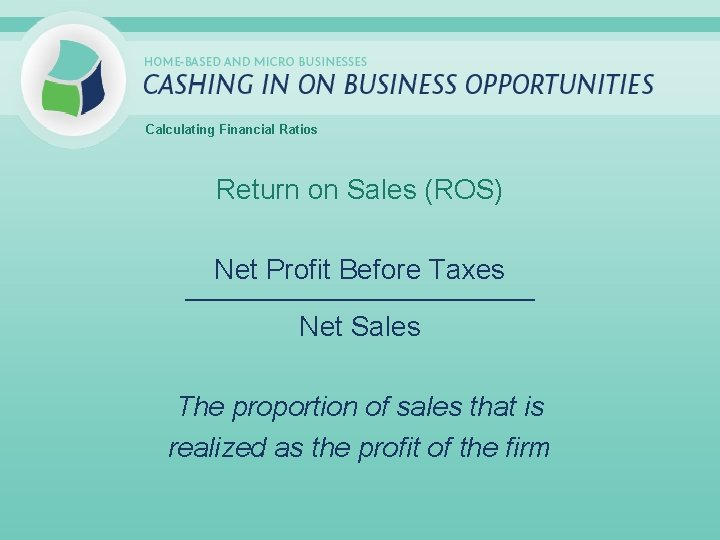Calculating Financial Ratios Return on Sales (ROS) Net Profit Before Taxes _____________________________ Net Sales The proportion of sales that is realized as the profit of the firm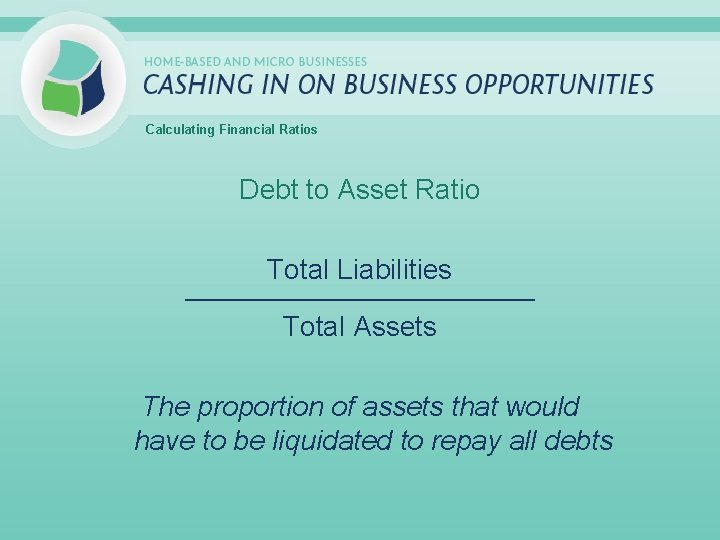Calculating Financial Ratios Debt to Asset Ratio Total Liabilities _____________________________ Total Assets The proportion of assets that would have to be liquidated to repay all debts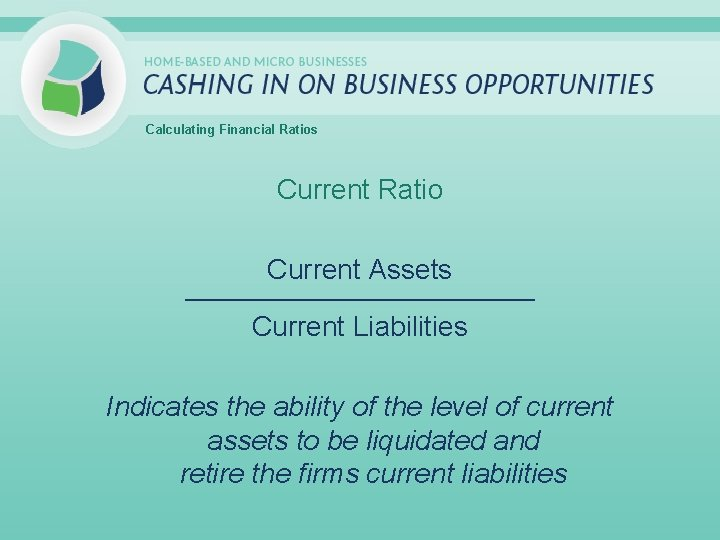Calculating Financial Ratios Current Ratio Current Assets _____________________________ Current Liabilities Indicates the ability of the level of current assets to be liquidated and retire the firms current liabilities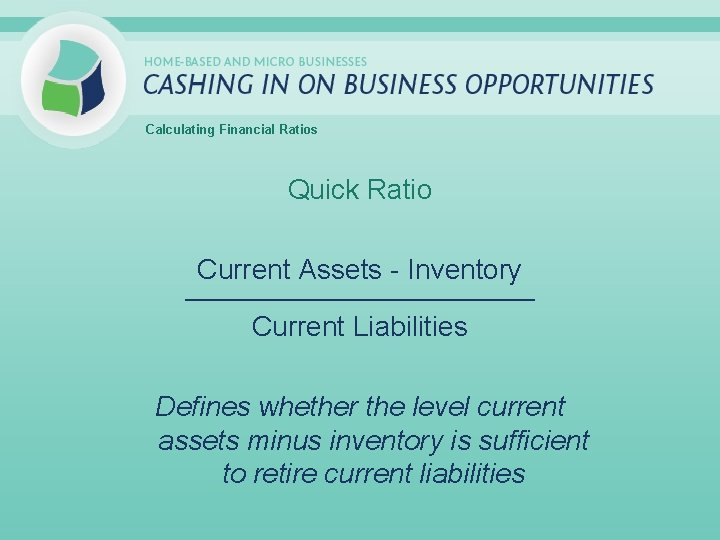Calculating Financial Ratios Quick Ratio Current Assets - Inventory _____________________________ Current Liabilities Defines whether the level current assets minus inventory is sufficient to retire current liabilities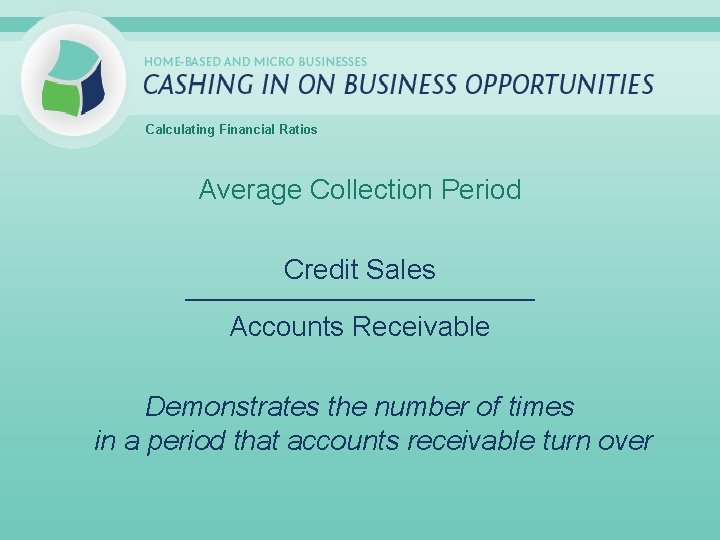Calculating Financial Ratios Average Collection Period Credit Sales _____________________________ Accounts Receivable Demonstrates the number of times in a period that accounts receivable turn over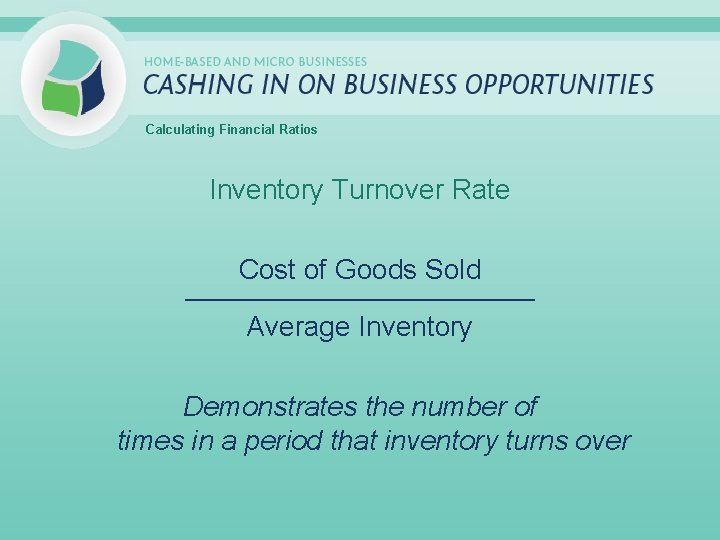Calculating Financial Ratios Inventory Turnover Rate Cost of Goods Sold _____________________________ Average Inventory Demonstrates the number of times in a period that inventory turns over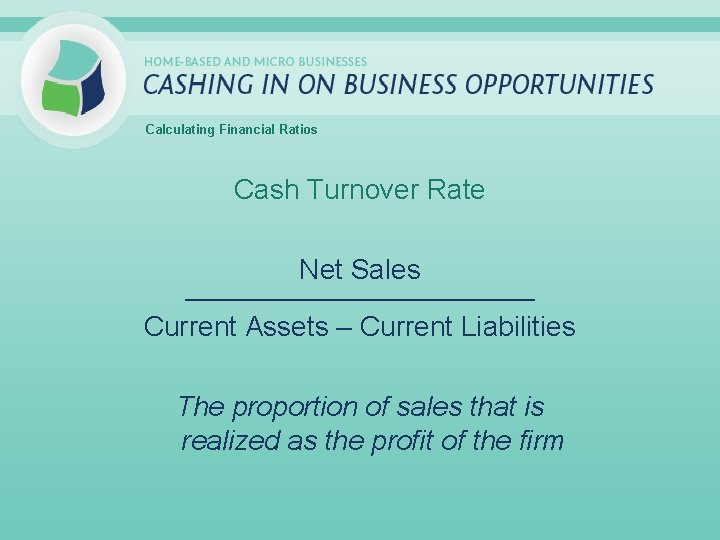Calculating Financial Ratios Cash Turnover Rate Net Sales _____________________________ Current Assets – Current Liabilities The proportion of sales that is realized as the profit of the firm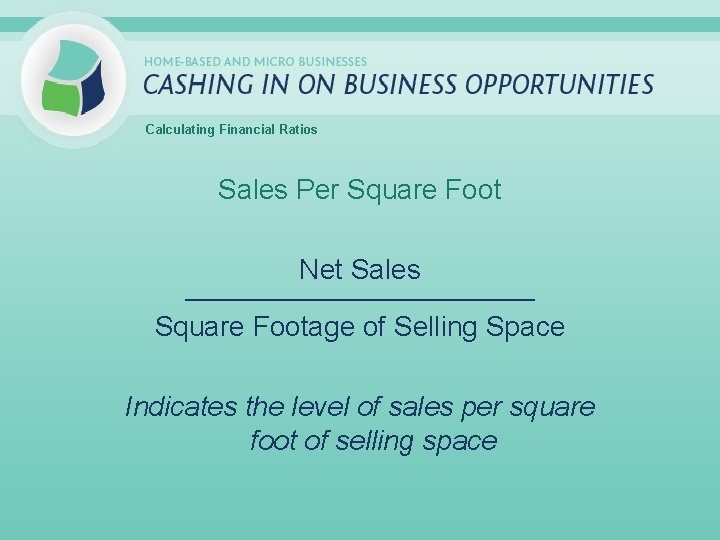Calculating Financial Ratios Sales Per Square Foot Net Sales _____________________________ Square Footage of Selling Space Indicates the level of sales per square foot of selling space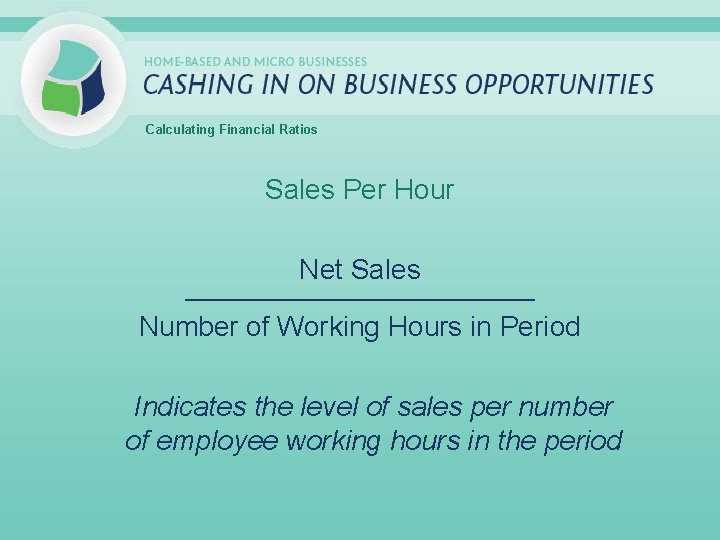Calculating Financial Ratios Sales Per Hour Net Sales _____________________________ Number of Working Hours in Period Indicates the level of sales per number of employee working hours in the period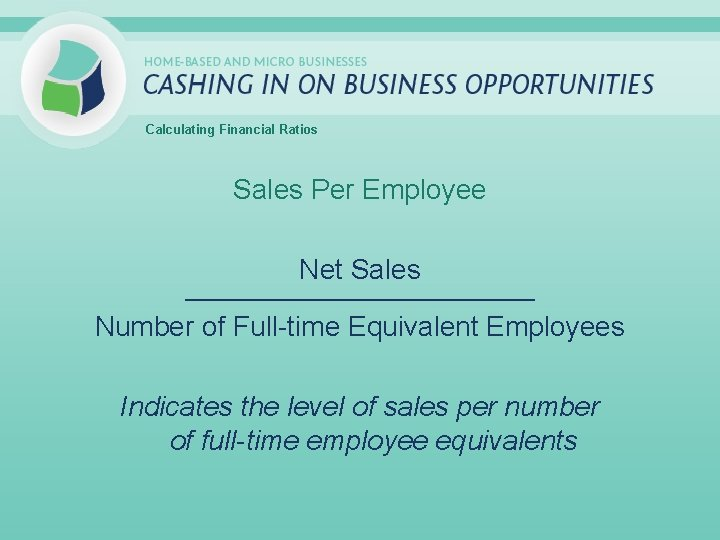Calculating Financial Ratios Sales Per Employee Net Sales _____________________________ Number of Full-time Equivalent Employees Indicates the level of sales per number of full-time employee equivalentsCalculating Financial Ratios Sales to Total Assets Net Sales _____________________________ Total Assets Indicates the level of sales per dollar of assets invested in the businessCalculating Financial Ratios Ratio Analysis Process 1. Calculate ratios 2. Maintain records of your ratios over time 3. Compare your ratios against similar businesses 4. Determine where adjustment is needed and develop action strategies 5. Measure results